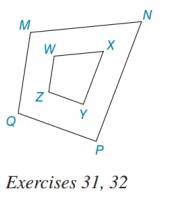Chapter 5.1, Problem 31E### Elementary Geometry for College St...

6th Edition
Daniel C. Alexander + 1 other
ISBN: 9781285195698

#### Solutions

Chapter
Section### Elementary Geometry for College St...

6th Edition
Daniel C. Alexander + 1 other
ISBN: 9781285195698
Textbook Problem
3 views

# For the quadrilaterals shown, M N W X = N P X Y = P Q Y Z = M Q W Z . If MN = 7, WX = 3, and PQ = 6, to find YZ.To determine

To find:

The value of YZ.

Explanation

According to Means-Extremes Property, in a proportion, the product of the means equals the product of the extremes.

Calculation:

It is given that MNWX=NPXY=PQYZ=MQWZ, MN = 7, WX = 3 and PQ = 6

Consider, MNWX=PQYZ

Substitute MN = 7, WX = 3 and PQ = 6 in MNWX=PQYZ

### Still sussing out bartleby?

Check out a sample textbook solution.

See a sample solution

#### The Solution to Your Study Problems

Bartleby provides explanations to thousands of textbook problems written by our experts, many with advanced degrees!

Get Started